## ISC Chemistry Previous Year Question Paper 2016 Solved for Class 12

Maximum Marks: 70
Time allowed: 3 hours

• Answer all questions in Part I and six questions from Part II, choosing two questions from Section A, two from Section B and two from Section C.
• All working, including rough work, should be done on the same sheet as, and adjacent to, the rest of the answer.
• The intended marks for questions or parts of questions are given in brackets [ ].
• Balanced equations must be given wherever possible and diagrams where they are helpful.
• When solving numerical problems, all essential working must be shown.
• In working out problems use the following data:
Gas constant R = 1.987 cal deg-1 mol-1 = 8.314 JK-1 mol-1 = 0.0821 dm3 atm K-1 mol-1. 1 L atm = 1 dm3 atm = 101.3 J.

Part – I (20 Marks)

Question 1.
(a) Fill in the blanks by choosing the appropriate word/words from those given in the brackets: 
(Henry’s, aldol condensation, absence, do not, ohm, Raoult’s, increases, common ion effect, easily, three, solubility product, ohm-1, two, four, ohm-1, cm2, Cannizzaro, ohm-1 cm-1, zero, decreases, presence)
(i) Ideal solutions obey ………. law and they …………. form azeotropic mixtures.
(ii) Benzaldehyde undergoes ……….. reaction due to ………… of an α-hydrogen atom.
(iii) The solubility of silver chloride ……… in the presence of sodium chloride because of ……….
(iv) The unit of conductance is ………. and that of specific conductance is ………..
(v) When the concentration of a reactant of the first-order reaction is doubled, the rate becomes ……… times, but for ……….. order reaction, the rate remains the same.

(b) Complete the following statements by selecting the correct alternative from the choices given: 
(i) The electrochemical equivalent is the amount of substance which gets deposited from its solution on passing electrical charge equal to:
(1) 96,500 Coulombs
(2) 1 Coulomb
(3) 60 Coulombs
(4) 965 Coulombs

(ii) The complexion [Ni(CN)4]2- is:
(1) Square planar and diamagnetic
(2) Tetrahedral and Paramagnetic
(3) Square planar and paramagnetic
(4) Tetrahedral and Diamagnetic

(iii) Wohler’s synthesis is used for the preparation of:
(1) Glycine
(2) Amino acids
(3) Urea
(4) Proteins

(iv) When SO2 gas is passed through the acidified K2Cr2O7 solution, the colour of the solution changes to:
(1) Red
(2) Black
(3) Orange
(4) Green

(v) In the equation $$\mathrm{CH}_{3} \mathrm{COOH}+\mathrm{Cl}_{2} \frac{\mathrm{Red} P}{-\mathrm{HCl}} \mathrm{A}$$, the compound A is:
(1) CH3CH2Cl
(2) ClCH2COOH
(3) CH3Cl
(4) CH3COCl

(c) Answer the following questions: 
(i) What is the order of reaction whose rate constant has the same unit as the rate of reaction?
(ii) What is the pH value of a solution whose hydroxyl ion concentration is 1 × 10-2 M?
(iii) Calculate the number of coulombs required to deposit 5.4 g of Al when the electrode reaction
is: Al3 + 3e + Al [Atomic Weight of Al 27 g/mol].
(iv) Write the reaction to prepare acetaldehyde from hydrogen gas and acid chloride.
(v) The edge length of the unit cell of a body-centred cubic (bcc) crystal is 352 pm. Calculate the radius of the atom.

(d) Match the following: 

 (i) Weak electrolyte (a) pH of a solution (ii) Colour in crystals (b) Iodoform (iii) Acetone (c) Tollen’s reagent (iv) Sorensen (d) Ostwald dilution law (v) Ammoniacal silver nitrate (e) F – centre

(a) (i) Raoult’s, do not
(ii) Cannizzaro, absence
(iii) decreases, common ion effect
(iv) ohm-1, ohm-1, cm-1
(v) two, zero

(b) (i) -1
(ii) -1
(iii) -3
(iv) -4
(v) -2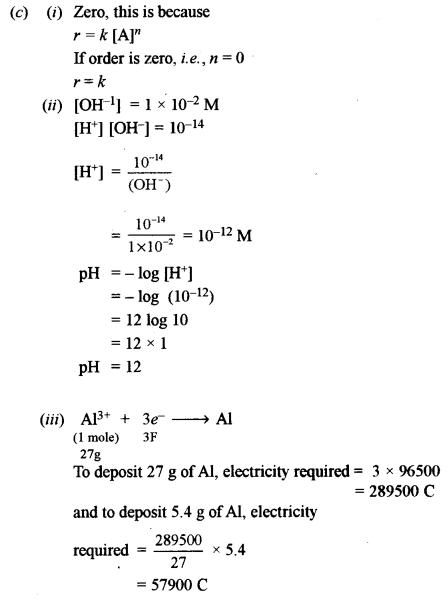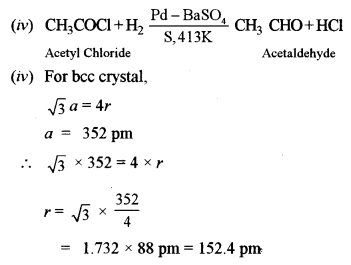(d) (i) (d)
(ii) (e)
(iii) (b)
(iv) (a)
(v) (c)

Part – II (50 Marks)

A nswer six questions choosing two from Section A, two from Section B and two from Section C.

Section – A

Question 2.
(a) (i) A10% aqueous solution of cane sugar (mol. wt. 342) is isotonic with 1.754% aqueous solution of urea. Find the molecular mass of urea. 
(ii) The molecular weight of an organic compound is 58 g mol-1. What will be the boiling point of a solution containing 48 grams of the solute in 1200 grams of water? 
[Kb for water = 0.513°C kg mole-1; Boiling point of water = 100°C]
(iii) What will be the value of Van’t Hoff factor (i) of benzoic acid if it dimerises in aqueous solution? How will the experimental molecular weight vary as compared to the normal molecular weight? 
(b) (i) Determine thepHvalueof 0.001 M acetic acid solution if it is 2% ionised at this concentration. How can the degree of dissociation of this acetic acid solution be increased? 
(ii) The solubility product of PbCl2 at 298K is 1.7 × 10-5. Calculate the solubility of PbCl2 in g/lit. at 298K. 
Atomic Weights: [Pb = 207 and Cl = 35.5]
(c) Graphite is anisotropic with respect to the conduction of electric current. Explain. 
(a) (i) 10% aqueous solution of cane sugar is isotonic with 1.754% aqueous solution of urea (MB)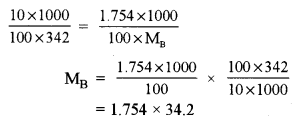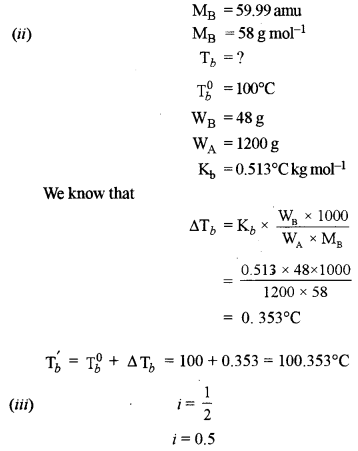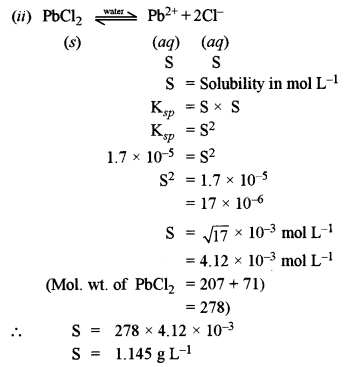(c) This is due to its chemical bonding properties. In graphite, there are C-atoms arranged in hexagonal layers which are held together by weak van der Waals forces of attraction.

Question 3.
(a) (i) In a body centred and face centred arrangement of atoms of an element, what will be the number of atoms present in respective unit cells? Justify your answer with a calculation. 
(ii) A compound AB has a simple cubic structure and has molecular mass 99. Its density is 3.4 g cm-3. What will be the edge length of the unit cell? 
(b) (i) For the reaction:
$$\begin{array}{c}{2 \mathrm{NO}_{(\mathrm{g})} \rightleftharpoons \mathrm{N}_{2(\mathrm{g})}+\mathrm{O}_{2(\mathrm{g})} ; \Delta \mathrm{H}=-\text { heat }} \\ {\mathrm{K}_{\mathrm{e}}=2.5 \times 10^{2} \mathrm{at} 298 \mathrm{K}}\end{array}$$
what will happen to the concentration of N2 if:
(1) Temperature is decreased to 273 K.
(2) The pressure is reduced
(ii) In a first-order reaction, 10% of the reactant is consumed in 25 minutes. Calculate: 
(1) The half-life period of the reaction.
(2) The time required for completing 87.5% of the reaction.
(c) Water acts as Bronsted acid as well as a Bronsted base. Give one example each to illustrate this statement. 
(a) (i) In bed unit cell, the atoms are present only at the comers of a cube.
Total number of atoms in one unit cell = $$8 \times \frac{1}{8}$$ = 1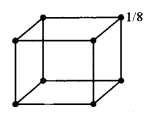In face centred cubic unit cell,
The atoms are present at the comers as well as at the centres of six faces of a cube.
Total number of atoms in one unit cell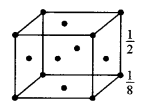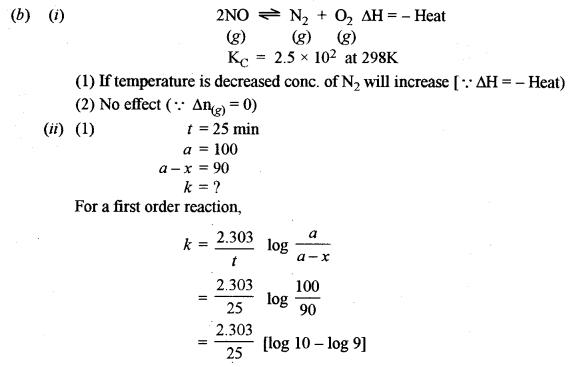Question 4.
(a) (i) Consider the following cell reaction at 298 K: 
2Ag+ + Cd → 2Ag + Cd2+
The standard reduction potentials (E°) for Ag+/Ag and Cd2-/Cd are 0.80 V and -0.40 V respectively:
(1) Write the cell representation.
(2) What will be the emf of the cell if the concentration of Cd2+ is 0.1M and that of Ag+ is 0.2 M?
(3) Will the cell work spontaneously for the condition given in (2) above?
(ii) What is a buffer solution? How is it prepared? Explain the buffer action of a basic buffer with a suitable example. 
(b) Explain the following: 
(i) When NaCl is added to AgNO3 solution, a white precipitate is formed.
(ii) An aqueous solution of ammonium chloride is acidic in nature.
(c) A 0.05 M NH4OH solution offers the resistance of 50 ohms to a conductivity cell at 298 K. If the cell constant is 0.50 cm-1 and molar conductance of NH4OH at infinite dilution is 471.4 ohm-1 cm2 mol-1, calculate:
(i) Specific conductance
(ii) Molar conductance
(iii) Degree of dissociation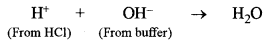(3) Since Ecell is +ve, the cell will work spontaneously.
(ii) Buffer solution: It is a solution whose pH remains unchanged on the addition of a small amount of acid or base or on dilution.
Preparation of buffer solution: A buffer solution can be prepared by mixing weak acid and its salt with a strong base or a weak base and its salt with the strong acid.
The action of a basic buffer
Let us consider a basic buffer obtained by mixing solutions containing 1 mole of NH4OH and 1 mole of NH4Cl. Its pH value is nearly 9.25.
In the solution there will be a large concentration of $$\mathrm{NH}_{4}^{+}$$ and Cl ions and unionised NH4OH molecules.

(a) Suppose a few drops of HCl are added to the buffer. Here H+ ions from HCl will combine with OH ions from NH4OH to form unionised water molecules.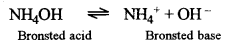According to Le-Chatelier’s principle, due to the removal of OH ions, the equilibrium (1) will shift towards r.h.s., giving more of OH ions and original concentration of OH ions is restored. Therefore, pH of the solution remains unchanged.

(b) Suppose a few drops of NaOH are added to the buffer. Here OH ions from NaOH combine with NH4+ ions from NH4Cl to give weakly ionised NH4OH molecules.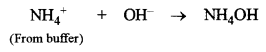(c) Suppose the buffer solution is diluted with water, it will not change the pH of the solution because the concentration of H+ and OH ions remains unchanged.

(b) (i) When NaCl is added to AgNO3, a white precipitate of AgCl is formed due to the combination of Cl ions from NaCl with Ag+ ions from AgNO3.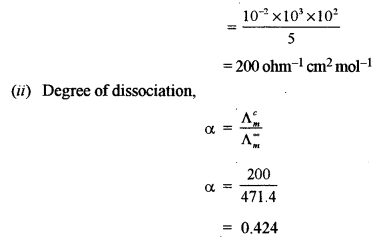Section – B

Question 5.
(a) Write the IUPAC names of the following: 
(i) [CO(NH3)4SO4]NO3
(ii) K[Pt(NH3)Cl3]
(b) What type of isomerism is exhibited by the following pairs of compounds: 
(i) [PtCl2(NH3)4]Br2 and [PtBr2(NH3)4]Cl2
(ii) [Cr(SCN)(H2O)5]2+ and [Cr(NCS)(H2O)5]2+
(c) How does K2[PtCl4] get ionised when dissolved in water? Will it form a precipitate when AgNO3 solution is added to it? Give a reason for your answer. 
(a) [CO(NH3)5 SO4] NO3
penta ammine sulphato cobalt (III) nitrate
K [Pt (NH3) Cl3]
potassium ammine tri chlorido platinate (II)

(b) (i) Ionisation isomerism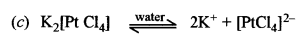It gives no chloride ion in solution, therefore, it will not form a precipitate with AgNO3 solution.

Question 6.
(a) Give balanced equations for the following reactions: 
(i) Silver nitrate is added to the dilute solution of sodium thiosulphate.
(ii) Potassium dichromate is treated with acidified ferrous sulphate solution.
(iii) Phosphorus reacts with cone, sulphuric acid.
(b) How will you obtain pure potassium permanganate (KMnO4) crystals from its ore, pyrolusite? Give the steps involved and the reactions.(b) Potassium permanganate crystals (KMnO4) from pyrolusite:
It involves two steps
Step-1: Conversion of pyrolusite into potassium manganate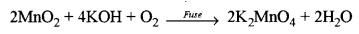Step-2: Conversion of potassium manganate into potassium permanganate (By isolation)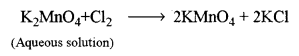On crystallisation, the crystals of KMnO4 appear, which are separated and dried.

Question 7.
(a) (i) Sulphur dioxide acts as an oxidizing agent as well as a reducing agent. Give one reaction each to show its oxidizing nature and its reducing nature. 
(ii) Explain why an aqueous solution of potassium hexacyanoferrate (II) does not give the test for ferrous ion. 
(b) What is meant by Lanthanide contraction? Write the general electronic configuration of inner transition elements. 
(a) (i) 1. SO2 as an oxidising agent
2Mg + SO2 → 2MgO + S
(Burning)
2. SO2 as a reducing agent
SO2 + Br2 + 2H2O → H2SO4 + 2HBr
(ii) Potassium hexacyanoferrate (II) does not give ferrous (Fe2+) ions in solution because it is complex and Fe2+ ions are in the coordination sphere and form coordinate bonds with cyanide, CN ligands.

(b) Lanthanide contraction
It is the slow or gradual decrease in the sizes of atoms or tri positive ions of lanthanide as we move from left to right along the lanthanides.
General electronic configuration of inner transition elements is
[Xe]54 4f1-14 5d0-1 6s2 – For lanthanides
[Rn]86 5f1-14 6d0-1 7s2 – For actinides

Section – C

Question 8.
(a) How can the following conversions be brought about:
(i) Acetaldehyde to acetaldehyde phenyl hydrazone. 
(ii) Benzoic acid to aniline. 
(iii) Methyl chloride to acetone. 
(iv) Benzene to benzene diazonium chloride. 
(b) (i) Glycerol (propane 1, 2, 3 triol) is more viscous than ethylene glycol (ethane 1, 2, diol). Explain 
(ii) How can urea be detected by Biuret test? 
(c) Identify the compounds A, B and C: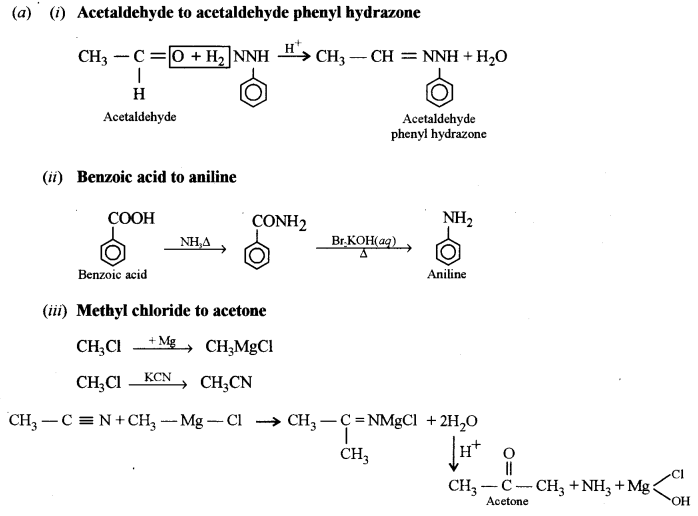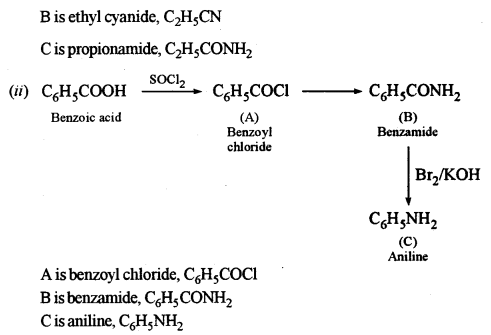Question 9.
(a) Give balanced equations for the following name reactions:
(i) Benzoin condensation
(ii) Wurtz-Fitting reaction
(iii) Carbylamine reaction
(b) Give the chemical test to distinguish:
(i) Formaldehyde and acetaldehyde
(ii) Dimethyl ether and ethyl alcohol.
(c) (i) Write the structure of three ethers with molecular formula C4H10O.
(ii) Starting with Grignard’s reagent, how will you prepare propanoic acid?(b) Distinction between:
(i) Formaldehyde and acetaldehyde
Acetaldehyde, when heated with I2 and NaOH, gives a yellow precipitate of iodoform (Iodoform test) whereas formaldehyde does not give a yellow precipitate of iodoform.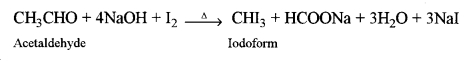(ii) Dimethyl ether and ethyl alcohol: C2H5OH, when heated with I2and NaOH, gives yellow precipitate crystals of iodoform (Iodoform test) whereas diethyl ether does not give a yellow precipitate with I2 and NaOH.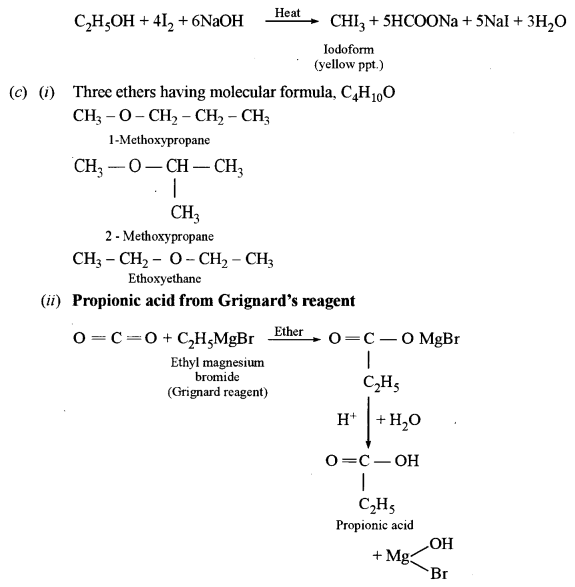Question 10.
(a) An organic compound A has the molecular formula C7H6O. When A is treated with NaOH followed by acid hydrolysis, it gives two products B and C. When B is oxidized, it gives A when A and C are each treated separately with PCl5, they give two different products D and E. 
(i) Identify A, B, C, D and E.
(ii) Give the chemical reaction when A is treated with NaOH and name the reaction.
(i) What do you observe when glucose solution is heated with Tollen’s reagent?
(ii) Name the monomers and the type of polymerisation in each of the following polymers:
(1) Terylene
(2) Polyvinylchloride
(c) Give balanced equations for the following reactions: 
(i) Ethylamine with nitrous acid.
(ii) Diethyl ether with phosphorus pentachloride.
(iii) Aniline with acetyl chloride.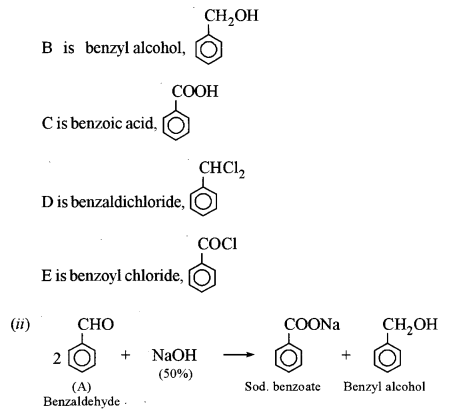This reaction is called Cannizzaro’s reaction.

(b) (i) When glucose solution is heated with Tollen’s reagent in a test tube, metallic silver gets deposited along the walls of a test tube and it shines like a silver mirror. It is called the silver mirror test or Tollen’s reagent test.
(ii) Monomers of
(1) Terylene is Terephthalic acid and Ethylene glycol
It involves condensation polymerisation
(2) Polyvinyl chloride is vinyl chloride